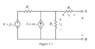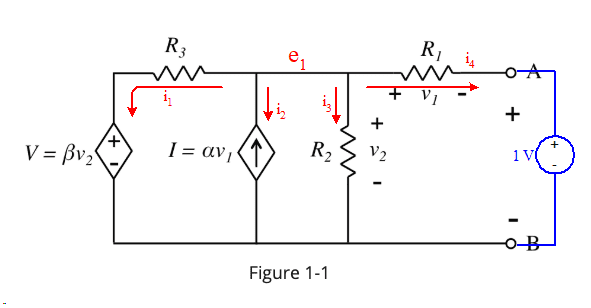# Finding the Thevenin equivalent Resistance for circuit with voltage dependent sources

• Engineering
JessicaHelena
Homework Statement:
A linear circuit containing two voltage dependent sources, as shown. The elements in this circuit have the following values: ##R_1 = 7.2k## Ohms, ##R_2 = 12k## Ohms, ##R_3 = 3.3k## Ohms, ##\alpha = 0.025## A/V and ##\beta = 5##.

Calculate the numerical value for the Thevenin equivalent resistance for terminal A-B.
Relevant Equations:
KVL and KCL
I am having a hard time solving this. Letting \$i\$ be the current flowing into ##R_3##, ##i_1## the current flowing through ##R_1## and ##i_2## the current through ##R_1##—and the node between R3 and R1 be e_1;

Using KVL and KCL, I've managed to find that

##i + I = i_1 + i_2##
Hence ##\frac{e_1-\beta v_2}{R_3} + \alpha v_1 = \frac{v_2}{R_2} + \frac{v_1}{R_1}##

also from one of the loops around V, R3 and R2, I have ##e_1 - 2\beta v_2 + v_2 = 0##.

I have 3 unknowns so far, so I'm trying to find another equation, but I'm not sure what to do from here.

#### Attachments

•Screenshot 2020-01-12 at 8.35.03 PM.png
4.7 KB · Views: 201

Mentor
Note that there are no independent sources in the circuit. So the whole thing will be inert without some outside stimulation.

You'll have to place a source at A-B to activate the circuit.

JessicaHelena
@gneill
Could I just choose any source? I heard 1 Volt or 1 Amp is common, but I'm not sure which is the better choice.

Mentor
@gneill
Could I just choose any source? I heard 1 Volt or 1 Amp is common, but I'm not sure which is the better choice.
Yes, you can choose any source of any value. Sometimes the circuit may be easier to analyze with a voltage source or with a current source, it depends upon the details of the circuit and your preferred analysis "tool kit". It'll basically be down to your intuition to choose one over the other. 1 Volt or 1 Amp are both easy to manipulate in equations, so they're a common choice for the external source.

JessicaHelena
@gneill

Couldn't I just short out the voltage source and open the current source? Is it because they're dependent?

JessicaHelena
would I also be right in thinking that v2 = vTH here?

Mentor
@gneill

Couldn't I just short out the voltage source and open the current source? Is it because they're dependent?
Yes, you cannot suppress dependent sources. They will have an active role in determining the impedance of the circuit (Thevenin resistance).

JessicaHelena
@gneill

Okay, so here's my (incomplete) approach.

I put V=1 volt across A and B. Hence v1 = v2 - 1.
From KCL at the node between R3 and R1, I also have that ##\frac{\beta v_2}{R_3} + \alpha v_1 + \frac{v_2}{R_2} + \frac{v_1}{R_1} = 0##

This is equivalent to ##\frac{\beta v_2}{R_3} + \alpha (v_2 - 1) + \frac{v_2}{R_2} + \frac{v_2 - 1}{R_1} = 0##

This gives me ##v_2 = 0.940215##, which does give me resistance across the current source ##I=\alpha v_1##, but I'm not really sure what else to do and I don't think that is the answer.

Mentor
You have some problems with your KCL (nodal analysis) equation. You need to decide whether you're summing currents flowing into the node or currents flowing out of the node, and be consistent in applying one or the other.

Call your node voltage ##e_1## as you did before. Then, summing currents flowing INTO that node, your first term would be:

$$\frac{\beta v_2 - e_1}{R_3}$$
Note that ##e_1 = v_2##, so plugging in known values that first term becomes:
$$\frac{5 e_1 - e_1}{3300} = \frac{4 e_1}{3300}$$

JessicaHelena
@gneill From the way the diagram is drawn, I thought that all the currents were going into node ##e_1##—from what I understand, current flows out of positive terminals. That's why I summed everything up. Is this wrong?

JessicaHelena
With your correction above, I got that ##v_2 = e_1 = 1##. That makes ##v_1 = 0##. And without voltage there, I think ##R_1## can then be ignored (bc no voltage means short circuit?).

Then I have R2 and R3 in parallel, which gives me R_eff = R_TH (is this right?) = 2.58824 k Ohms.

Mentor
@gneill From the way the diagram is drawn, I thought that all the currents were going into node ##e_1##—from what I understand, current flows out of positive terminals. That's why I summed everything up. Is this wrong?
Best not to try to determine the current directions or potential values ahead of time. When you have current sources that define the direction, that's fine. Otherwise let the math take care of it for you.

Let's take a detailed look at writing the node equation for your circuit. I'll choose to sum currents flowing OUT of the node. Labeling the currents involved:So for ##i_1## we have:

##i_1 = \frac{e_1 - 5 e_1}{3300}##

##i_2## is forced to flow INTO the node by the dependent current source, and that's opposite the direction of the labeled ##i_2##. So this term becomes negative:

##i_2 = - \alpha v_1##

Note that ##\alpha = 0.025 = 1/40## and that ##v_1 = e_1 - 1##. So that gives us:

##i_2 = - \frac{e_1 - 1}{40}##

##i_3## is simple:

##i_3 = \frac{e_1}{12000}##

Finally, for ##i_4##

##i_4 = \frac{e_1 - 1}{7200}##

Sum them up and solve for ##e_1##.

Mentor
Then I have R2 and R3 in parallel, which gives me R_eff = R_TH (is this right?) = 2.58824 k Ohms.
Nope. You cannot suppress dependent sources to simplify the circuit. That's a definite no-no.

JessicaHelena
@gneill
Hmm. so from your help in post #12, I calculated ##e_1 = 0.956568 = v_2##. Since ##v_1 = v_2 - 1##, I calculate ##v_1 = -0.043432##. The negative tells us that ##i_4## actually flows in the opposite direction, towards ##e_1##. ( In fact, except for i3, all of the currents actually have an opposite direction to the ones depicted. )

The current flowing directly into the rest of the circuit from the battery is ##i_4 = -6.0322*10^(-6)##...

Or should I just use ##v_2## divided by ##i_3## to get the resistance? I'm still a bit confused as to how to finish off the problem.

Mentor
@gneill
Hmm. so from your help in post #12, I calculated ##e_1 = 0.956568 = v_2##. Since ##v_1 = v_2 - 1##, I calculate ##v_1 = -0.043432##. The negative tells us that ##i_4## actually flows in the opposite direction, towards ##e_1##. ( In fact, except for i3, all of the currents actually have an opposite direction to the ones depicted. )
Yup. I purposely chose that to write the sum of currents flowing OUT of the node in order to demonstrate that in the end it doesn't matter whether you take all the currents to be flowing in or all the currents to be flowing out; the math automatically gives you a correct answer by adjusting the signs.
The current flowing directly into the rest of the circuit from the battery is ##i_4 = -6.0322*10^(-6)##...

Or should I just use ##v_2## divided by ##i_3## to get the resistance? I'm still a bit confused as to how to finish off the problem.
Since your battery is 1 V, the simplest is to write:

##R_{th} = \frac{1}{6.0322*10^{-6}}##

JessicaHelena
Thank you so much!